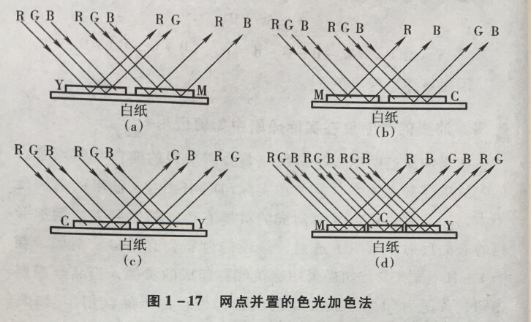# |

## 全部印刷品

 首页 >> 印刷专题 >>书刊印刷 >> 什么是书刊印刷网点并置呈色？

# 什么是书刊印刷网点并置呈色？

Y=R+G

M=B+R

Y+M=R+G+B+R=（R+G+B）+R=W+RM=B+R

C=G+B

M+C=B+R+G+B=B+（R+G+B）=B+W

C=G+B

Y=R+G

C+Y=G+B+R+G=（G+B+R）+G=W+G

Y=R+G

M=B+R

C=G+B

Y+M+C=R+G+B+R+G+B

=（R+G+B）+（R+G+B）

=W

# 关于美好视界 关于我们 联系我们 帮助中心

### 186 1663 4825# 操作视频

0
×

### 购物车